• 2021-08-21 18:16:47

## 前言

这篇文章LSTM 航空乘客预测 单步预测和多步预测。 简单运用LSTM 模型进行预测分析。
想着可以进一步改进LSTM模型，就采用了目前市面上比较流行的注意力机制，将两者进行结合，对LSTM进行改进，其预测效果可能会变好。

我喜欢直接代码+ 结果展示
先代码可以跑通，才值得深入研究每个部分之间的关系；进而改造成自己可用的数据。

## 1 数据集

参考上面文章，会找到数据集，这里不重复了。

## 2 模型

下载注意力机制模块

pip install attention


### 2.1 单步预测 1—》1

代码

# 单变量，1---》1

import numpy
import matplotlib.pyplot as plt
import math
from keras.models import Sequential
from keras.layers import Dense
from keras.layers import LSTM
from sklearn.preprocessing import MinMaxScaler
from sklearn.metrics import mean_squared_error
#matplotlib inline

# print(dataframe)
print("数据集的长度：",len(dataframe))
dataset = dataframe.values
# 将整型变为float
dataset = dataset.astype('float32')

plt.plot(dataset)
plt.show()

# X是给定时间(t)的乘客人数，Y是下一次(t + 1)的乘客人数。
# 将值数组转换为数据集矩阵,look_back是步长。
def create_dataset(dataset, look_back=1):
dataX, dataY = [], []
for i in range(len(dataset)-look_back-1):
a = dataset[i:(i+look_back), 0]
# X按照顺序取值
dataX.append(a)
# Y向后移动一位取值
dataY.append(dataset[i + look_back, 0])
return numpy.array(dataX), numpy.array(dataY)

# fix random seed for reproducibility
numpy.random.seed(7)

# 数据缩放
scaler = MinMaxScaler(feature_range=(0, 1))
dataset = scaler.fit_transform(dataset)

# 将数据拆分成训练和测试，2/3作为训练数据
train_size = int(len(dataset) * 0.67)
test_size = len(dataset) - train_size
train, test = dataset[0:train_size,:], dataset[train_size:len(dataset),:]
print("原始训练集的长度：",train_size)
print("原始测试集的长度：",test_size)

# 构建监督学习型数据
look_back = 1
trainX, trainY = create_dataset(train, look_back)
testX, testY = create_dataset(test, look_back)
print("转为监督学习，训练集数据长度：", len(trainX))
# print(trainX,trainY)
print("转为监督学习，测试集数据长度：",len(testX))
# print(testX, testY )
# 数据重构为3D [samples, time steps, features]
trainX = numpy.reshape(trainX, (trainX.shape, 1, trainX.shape))
testX = numpy.reshape(testX, (testX.shape, 1, testX.shape))
print('构造得到模型的输入数据(训练数据已有标签trainY): ',trainX.shape,testX.shape)

# create and fit the LSTM network
from attention import  Attention
model = Sequential()
Attention(name='attention_weight')
model.fit(trainX, trainY, epochs=100, batch_size=1, verbose=2)

# 打印模型
model.summary()

# 开始预测
trainPredict = model.predict(trainX)
testPredict = model.predict(testX)

# 逆缩放预测值
trainPredict = scaler.inverse_transform(trainPredict)
trainY = scaler.inverse_transform([trainY])
testPredict = scaler.inverse_transform(testPredict)
testY = scaler.inverse_transform([testY])

# 计算误差
trainScore = math.sqrt(mean_squared_error(trainY, trainPredict[:,0]))
print('Train Score: %.2f RMSE' % (trainScore))
testScore = math.sqrt(mean_squared_error(testY, testPredict[:,0]))
print('Test Score: %.2f RMSE' % (testScore))

# shift train predictions for plotting
trainPredictPlot = numpy.empty_like(dataset)
trainPredictPlot[:, :] = numpy.nan
trainPredictPlot[look_back:len(trainPredict)+look_back, :] = trainPredict

# shift test predictions for plotting
testPredictPlot = numpy.empty_like(dataset)
testPredictPlot[:, :] = numpy.nan
testPredictPlot[len(trainPredict)+(look_back*2)+1:len(dataset)-1, :] = testPredict

# plot baseline and predictions
plt.plot(scaler.inverse_transform(dataset))
plt.plot(trainPredictPlot)
plt.plot(testPredictPlot)
plt.show()


结果展示：

Train Score: 22.98 RMSE
Test Score: 48.30 RMSE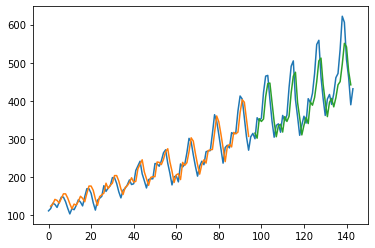预测下一个月的数据：

# 预测未来的数据

#测试数据的最后一个数据没有预测,这里补上
finalX = numpy.reshape(test[-1], (1, 1, testX.shape))

#预测得到标准化数据
featruePredict = model.predict(finalX)

#将标准化数据转换为人数
featruePredict = scaler.inverse_transform(featruePredict)

#原始数据是1949-1960年的数据,下一个月是1961年1月份
print('模型预测1961年1月份的国际航班人数是: ',featruePredict)


结果展示：

模型预测1961年1月份的国际航班人数是:  [[419.07907]]


### 2.2 单步预测 3—》1

代码：

# 单变量，3---》1

import numpy
import matplotlib.pyplot as plt
import math
from keras.models import Sequential
from keras.layers import Dense
from keras.layers import LSTM
from sklearn.preprocessing import MinMaxScaler
from sklearn.metrics import mean_squared_error
#matplotlib inline

# print(dataframe)
print("数据集的长度：",len(dataframe))
dataset = dataframe.values
# 将整型变为float
dataset = dataset.astype('float32')

plt.plot(dataset)
plt.show()

# X是给定时间(t)的乘客人数，Y是下一次(t + 1)的乘客人数。
# 将值数组转换为数据集矩阵,look_back是步长。
def create_dataset(dataset, look_back=1):
dataX, dataY = [], []
for i in range(len(dataset)-look_back-1):
a = dataset[i:(i+look_back), 0]
# X按照顺序取值
dataX.append(a)
# Y向后移动一位取值
dataY.append(dataset[i + look_back, 0])
return numpy.array(dataX), numpy.array(dataY)

# fix random seed for reproducibility
numpy.random.seed(7)

# 数据缩放
scaler = MinMaxScaler(feature_range=(0, 1))
dataset = scaler.fit_transform(dataset)

# 将数据拆分成训练和测试，2/3作为训练数据
train_size = int(len(dataset) * 0.67)
test_size = len(dataset) - train_size
train, test = dataset[0:train_size,:], dataset[train_size:len(dataset),:]
print("原始训练集的长度：",train_size)
print("原始测试集的长度：",test_size)

# 构建监督学习型数据
look_back = 3
trainX, trainY = create_dataset(train, look_back)
testX, testY = create_dataset(test, look_back)
print("转为监督学习，训练集数据长度：", len(trainX))
# print(trainX,trainY)
print("转为监督学习，测试集数据长度：",len(testX))
# print(testX, testY )
# 数据重构为3D [samples, time steps, features]
# trainX = numpy.reshape(trainX, (trainX.shape, 1, trainX.shape))
# testX = numpy.reshape(testX, (testX.shape, 1, testX.shape))

trainX = numpy.reshape(trainX, (trainX.shape,  trainX.shape,1))
testX = numpy.reshape(testX, (testX.shape, testX.shape, 1))

print('构造得到模型的输入数据(训练数据已有标签trainY): ',trainX.shape,testX.shape)

# create and fit the LSTM network
model = Sequential()

Attention(name='attention_weight')

model.fit(trainX, trainY, epochs=100, batch_size=1, verbose=2)

# 打印模型
model.summary()

# 开始预测
trainPredict = model.predict(trainX)
testPredict = model.predict(testX)

# 逆缩放预测值
trainPredict = scaler.inverse_transform(trainPredict)
trainY = scaler.inverse_transform([trainY])
testPredict = scaler.inverse_transform(testPredict)
testY = scaler.inverse_transform([testY])

# 计算误差
trainScore = math.sqrt(mean_squared_error(trainY, trainPredict[:,0]))
print('Train Score: %.2f RMSE' % (trainScore))
testScore = math.sqrt(mean_squared_error(testY, testPredict[:,0]))
print('Test Score: %.2f RMSE' % (testScore))

# shift train predictions for plotting
trainPredictPlot = numpy.empty_like(dataset)
trainPredictPlot[:, :] = numpy.nan
trainPredictPlot[look_back:len(trainPredict)+look_back, :] = trainPredict

# shift test predictions for plotting
testPredictPlot = numpy.empty_like(dataset)
testPredictPlot[:, :] = numpy.nan
testPredictPlot[len(trainPredict)+(look_back*2)+1:len(dataset)-1, :] = testPredict

# plot baseline and predictions
plt.plot(scaler.inverse_transform(dataset))
plt.plot(trainPredictPlot)
plt.plot(testPredictPlot)
plt.show()


结果展示：

Train Score: 28.57 RMSE
Test Score: 66.73 RMSE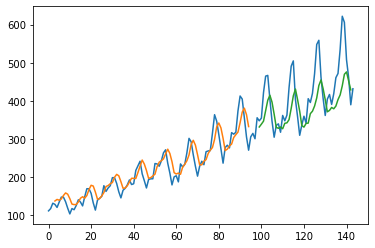预测下一个月的数据

# 预测未来的数据

#测试数据的最后一个数据没有预测,这里补上
finalX = numpy.reshape(test[-3:], (1, testX.shape, 1))
print(finalX)

#预测得到标准化数据
featruePredict = model.predict(finalX)

#将标准化数据转换为人数
featruePredict = scaler.inverse_transform(featruePredict)

#原始数据是1949-1960年的数据,下一个月是1961年1月份
print('模型预测1961年1月份的国际航班人数是: ',featruePredict)


结果展示

模型预测1961年1月份的国际航班人数是:  [[394.95465]]


## 3 总结

• 注意力机制的作用
• 代码中 LSTM后面连接注意力机制的代码
• 不是说加了注意力机制的LSTM网络一定会好于LSTM，具体要看数据、参数和实验环境等条件LSTM
更多相关内容
• import math import torch import torch.nn as nn import os def file_name_walk(file_dir): for root, dirs, files in os.walk(file_dir): # print(root, root) # 当前目录路径 print(dirs, dirs) # 当前路径下...
• 加入注意力机制的LSTM 对航空乘客预测_nsq1101的博客-CSDN博客.html
• 注意力机制诞生后，诞生了多种改进的模型，但是这些模型一般都是把注意力机制与循环神经网络结合（包括一些改良的，如LSTM），但是这些模型有个弊端：RNN由于其顺序结构训练速度常常受到限制，就是并行计算能力不...

### 引言

Transformer同样也是seq2seq模型。在注意力机制诞生后，诞生了多种改进的模型，但是这些模型一般都是把注意力机制与循环神经网络结合（包括一些改良的，如LSTM），但是这些模型有个弊端：RNN由于其顺序结构训练速度常常受到限制，就是并行计算能力不强。既然Attention模型本身可以看到全局的信息， 那么一个自然的疑问是我们能不能去掉RNN结构，仅仅依赖于Attention模型呢，这样我们可以使训练并行化（encoder端并行计算），同时拥有全局信息？为解决这一些问题，论文提出了一种仅基于注意力机制的模型，具有强大的并行计算性以及效果很好。

### 一、Structure of Transformers

Transformer模型的模型结构为encoder-decoder，如下图，N个Encoder block组成encoder，N个Decoder block组成decoder。基于注意力机制的构造与基于RNN的不同，基于RNN的是在时间步上串联（在每个time step只能输入一个token），而基于注意力机制的是类似于桶状结构（一起将数据输入到模型中去）。那么，在Transformer中encoder中的信息如何传给decoder？将encoder的最后一个Block分别传给decoder中的每一个block，然后decoder进行输出生成。

### 二、Encoder Structure以输入为：Thinking Machines为例，Thinking与Machines最开始输入是维度为vocab的one-hot向量，经过embedding层，转换成embedding size大小的向量，输入到self-Attention层中，得到 z z ，经过Feed Forward层将得到 r r r r 将作为下一个encoder Block的input。
其中，Self-attention将在后面详细分析，这里介绍FFN。每一层经过attention之后，还会有一个FFN，这个FFN的作用就是空间变换。FFN包含了2层linear transformation层，中间的激活函数是ReLu。FFN的加入引入了非线性(ReLu激活函数)，变换了attention output的空间, 从而增加了模型的表现能力。把FFN去掉模型也是可以用的，但是效果差了很多。

Word2vec的基本思想：句子中相近的词之间是有联系的，比如今天后面经常出现上午、下午和晚上。所以Word2vec的基本思想是用词来预测词，skip-gram使用中心词预测周围词，cbow使用周围词预测中心词。那么，一个词的embedding有没有可能关系到周围词外的部分？Self-attention就是自己与自己计算一个相关性（权重），利用自己（句子/文本）内部的表征得到一个更好的表征。#### 1.Self-attention

Self-Attention的基本结构如下：下面展示Self-attention内部是如何计算的Q Q :标的
K K :和别人计算的标的
V V :加权的vector标的
对于self-attention来讲, Q ( Q u e r y ) , K ( K e y ) , V ( V a l u e ) Q(Query), K(Key), V(Value) 三个矩阵均来自同一输入（Q、K、V来自embedding的线性变化），首先我们要计算 Q Q K K 之间的点乘，然后为了防止其结果过大，会除以一个尺度标度 d k \sqrt{d_k} ，其中 d k \sqrt{d_k} 为一个query和key向量的维度。再利用Softmax操作将其结果归一化为概率分布，然后再乘以矩阵 V V 就得到权重求和的表示。
举个例子：
假如我们要翻译一个词组Thinking Machines，其中Thinking的输入的embedding vector用 x 1 x_1 表示，Machines的embedding vector用 x 2 x_2 表示。当我们处理Thinking这个词时，我们需要计算句子中所有词与它的Attention Score，这就像将当前词作为搜索的query，去和句子中所有词（包含该词本身）的key去匹配，看看相关度有多高。我们用 q 1 q_1 代表Thinking对应的query vector， k 1 k_1 k 2 k_2 分别代表Thinking以及Machines对应的key vector，则计算Thinking的attention score的时候我们需要计算 q 1 q_1 k 1 , k 2 k_1,k_2 的点乘，同理，我们计算Machines的attention score的时候需要计算 q 2 q_2 k 1 , k 2 k_1,k_2 的点乘。我们分别得到了 q 1 q_1 k 1 , k 2 k_1,k_2 的点乘积，然后我们进行尺度缩放与softmax归一化，如下图所示：显然，当前单词与其自身的attention score一般最大，其他单词根据与当前单词重要程度有相应的score。然后我们在用这些attention score与value vector相乘，得到加权的向量。如果将输入的所有向量合并为矩阵形式，则所有query, key, value向量也可以合并为矩阵形式表示其中， W Q , W K , W V W^Q,W^K,W^V 是我们模型训练过程中学习到的合适参数，上述公式可以简化为矩阵计算：multi-head self-attention 与self-attention 的区别在于可以有多组 W Q , W K , W V W^Q,W^K,W^V ，得到不同的 Q 、 K 、 V Q、K、V ，最后得到不同的 Z Z 。我们认为一组线性变化提取的是一个维度的特征，采用多组可以从更高维来提取更多的特征。其中，多个self-attention得到的结果 Z i Z_i 后续操作如下所示：拼接后与矩阵 W o W^o 进行线性变化，### 四、Residuals与Layer-Normalization• Residuals:是为了解决多层神经网络训练困难的问题，通过将前一层的信息无差的传递到下一层，可以有效的仅关注差异部分，这一方法之前在图像处理结构如ResNet等中常常用到。 Transformer的层数较多，反向传播时容易出现梯度消失与梯度爆炸，所以添加Residuals，防止信息的丢失与梯度回传的消失
• Layer Normalization：在每个block中，最后出现的是Layer Normalization。Layer Normalization是一个通用的技术，其本质是规范优化空间，加速收敛。当我们使用梯度下降法做优化时，随着网络深度的增加，数据的分布会不断发生变化，为了保证数据特征分布的稳定性，我们加入Layer Normalization，这样可以加速模型的优化速度。

### 五、Decoder structure of Transformer#### 2.Encoder-decoder attentionEncoder-decoder attention的输入：它的Q来自于之前一级的decoder层的输出，但K和V来自于encoder的输出，这使得decoder的每一个位置都可以attend到输入序列的每一个位置。
下面展示Encoder-decoder attention的预测过程：### 六、Word Embedding and position encoding

通过前面encoder部分，我们可以发现：encoder端并行输入，没有了序列信息，此时，就需要position encoding。position encoding只在encoder端和decoder端的embedding之后，第一个block之前出现，它非常重要，没有这部分，Transformer模型就无法用。position encoding是Transformer框架中特有的组成部分，补充了Attention机制本身不能捕捉位置信息的缺陷。为了更好的让模型学习位置信息，所以添加了position encoding并将其叠加在word embedding上。该论文中选取了三角函数的encoding方式：p o s pos 表示的是在这句话的第几个位置， i i 表示的是在embedding的第几个维度。所以，通过三角函数，可以计算出这句话的每一个位置的每一个维度的值。这样计算的优势是：使模型轻松学到相对位置，因为对于任意的偏移量 k k P E p o s + k PE_{pos+k} 可以由 P E p o s PE_{pos} 的线性表示，同时，这个模型与可学习到的位置编码相比，可以解决sequence长度更长的位置信息。三角函数可视化结果为：注意：position encoding在训练过程中是固定的

### 七、扩展

为什么Transformer会work？

• Transformer做到内部之间的相互attention
• Transformer能够解决RNN/LSTM模型的长期依赖问题
• Transformer是大模型，有更多的参数，可以提取更高维的信息，学到更好的表征
• 利用self attention思想，结合预训练模型，能够产生语言学预训练知识，为后面的预训练模型提供了框架的基础，能方便下游任务做一个快速的finetune。

参考

如果对您有帮助，麻烦点赞关注，这真的对我很重要！！！如果需要互关，请评论或者私信！展开全文• 为解决泊位占有率的预测精度随步长增加而下降的问题，提出了一种基于注意力机制的泊位占有率预测模型。通过卷积神经网络获得多变量的时间模式信息作为模型的注意力机制。通过对模型训练、学习特征信息，并对相关性高...序列到序列模型
• 其次,基于双注意力模块,计算与轨迹生成关联度较高的个体运动信息和群体交互信息;最后,利用生成对抗网络进行全局联合训练,获得反向传播误差和各层的合理网络参数,解码器利用已获取的上下文信息生成多条合理预测轨迹。...
• 详解自注意力机制及其在LSTM中的应用 注意力机制（Attention Mechanism）最早出现在上世纪90年代，应用于计算机视觉领域。2014年，谷歌Mnih V等人 在图像分类中将注意力机制融合至RNN中，取得了令人瞩目的成绩，...

## 详解自注意力机制及其在LSTM中的应用

注意力机制（Attention Mechanism）最早出现在上世纪90年代，应用于计算机视觉领域。2014年，谷歌Mnih V等人 在图像分类中将注意力机制融合至RNN中，取得了令人瞩目的成绩，随后注意力机制也开始在深度学习领域受到广泛关注，在自然语言处理领域，Bahdanau等人 将注意力机制融合至编码-解码器中，在翻译任务取得不错的效果。而真正让注意力机制大火的是2017年，谷歌提出的Transformer，它提出了自注意力机制（self-Attention Mechanism），摒弃了RNN和CNN，充分挖掘了DNN的特性，刷新了11项NLP任务的精度，震惊了深度学习领域。
注意力机制基于人类的视觉注意力，人在观察物体的时候往往会把重点放在部分特征上，注意力机制就是根据这个特点，基于我们的目标，给强特征给予更大的权重，而弱特征给予较小权重，甚至0权重。

## 1.注意力机制本质

注意力机制（Attention Mechanism）的本质是：对于给定目标，通过生成一个权重系数对输入进行加权求和，来识别输入中哪些特征对于目标是重要的，哪些特征是不重要的；
为了实现注意力机制，我们将输入的原始数据看作< Key, Value>键值对的形式，根据给定的任务目标中的查询值 Query 计算 Key 与 Query 之间的相似系数，可以得到Value值对应的权重系数, 之后再用权重系数对 Value 值进行加权求和, 即可得到输出。我们使用Q,K,V分别表示Query, Key和Value，注意力权重系数W的公式如下:
W = s o f t m a x ⁡ ( Q K T ) W =softmax⁡(QK^T )
将注意力权重系数W与Value做点积操作（加权求和）得到融合了注意力的输出：
A t t e n t i o n ( Q , K , V ) = W ⋅ V = s o f t m a x ⁡ ( Q K T ) ⋅ V Attention(Q,K,V) = W·V=softmax⁡(QK^T )·V
注意力模型的详细结构如下图所示：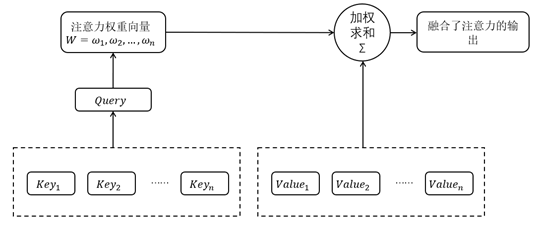需要注意，如果Value是向量的话，加权求和的过程中是对向量进行加权，最后得到的输出也是一个向量。
可以看到，注意力机制可以通过对< Key, Query>的计算来形成一个注意力权重向量，然后对Value进行加权求和得到融合了注意力的全新输出，注意力机制在深度学习各个领域都有很多的应用。不过需要注意的是，注意力并不是一个统一的模型，它只是一个机制，在不同的应用领域，Query, Key和Value有不同的来源方式，也就是说不同领域有不同的实现方法。

## 2.自注意力机制

自注意力机制（self-Attention Mechanism），它最早由谷歌团队在2017年提出，并应用于Transformer语言模型。自注意力机制可以在编码或解码中单独使用，相对于注意力机制，它更关注输入内部的联系，区别就是Q,K和V来自同一个数据源，也就是说Q,K和V由同一个矩阵通过不同的线性变换而来。
比如对于文本矩阵来说，利用自注意力机制可以实现文本内各词“互相注意”，即词与词之间产生注意力权重矩阵，然后对Value加权求和产生一个融合了自注意力的新文本矩阵。文本自注意力的实现步骤如下：

1. 假设文本矩阵 i n p u t = R ( a × b ) input=R^{(a×b)} ，三个变换矩阵（卷积核）： ω q , ω k ∈ R ( b × d ) 、 ω v ∈ R ( b × c ) ω^q,ω^k∈ R^{(b×d)}、ω^v∈ R^{(b×c)}
2. Q、K、V变换：文本矩阵和三个权重矩阵做线性变换，得到 Q , K ∈ R ( a × d ) 、 V ∈ R ( a × c ) Q,K∈ R^{(a×d)}、V∈ R^{(a×c)} :
Q = i n p u t ω q , K = i n p u t ω k , V = i n p u t ω v Q =input ω^q, K =input ω^k, V =input ω^v
3. 缩放点积： Q × K T Q×K^T 然后乘以一个 1 / d k 1/\sqrt{d_k} d k d_k 为K的维度， 1 / d k 1/\sqrt{d_k} 为缩放因子，防止内积数值过大影响神经网络的学习），得到注意力得分矩阵 G ∈ R ( a × a ) G∈ R^{(a×a)} ，G的行表示某个词在各个词上的得分：
G = Q K T / d k G =QK^T/\sqrt{d_k}
4. 得到注意力权重矩阵： s o f t m a x ( G ) softmax(G) 表示注意力权重矩阵W ：
W = s o f t m a x ( ( Q K T ) / d k ) W=softmax((QK^T)/\sqrt{d_k})
5. 得到结果矩阵： W* V 得到一个结果矩阵Attention∈ R^(a×c)，该矩阵就是一个全新的融合了注意力机制的文本矩阵z:
z = A t t e n t i o n ( Q , K , V ) = s o f t m a x ( Q K T / d k ) V z=Attention(Q,K,V) =softmax(QK^T/\sqrt{d_k})V

在上述公式中，变换矩阵 ω q 、 ω k 、 ω v ω^q、ω^k、ω^v 都是神经网络的参数，可以随着反向传播而修改，通过修改这些变换矩阵来达到自注意力转移的目的。

## 3.多头自注意力

若为多头自注意力机制，则有多组卷积核 ω i q , ω i v , ω i k ω_i^q,ω_i^v,ω_i^k ，将步骤2-5进行h次得到h组结果矩阵 ( z 1 , . . . , z h ) (z_1,...,z_h ) ，将 ( z 1 , . . . , z h ) (z_1,...,z_h ) 拼接并做一次线性变换 ω z ω^z 就得到了我们想要的文本矩阵：
M u l t i H e a d ( Q , K , V ) = C o n c a t ( z 1 , . . . , z h ) ω z MultiHead(Q,K,V )= Concat(z_1,...,z_h ) ω^z
缩放点积计算和多头自注意力机制计算过程如下图：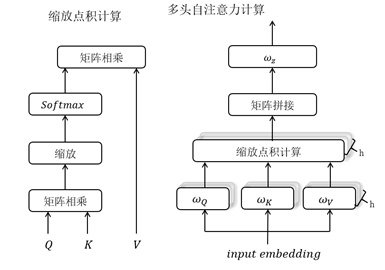自注意力机制将文本输入视为一个矩阵，没有考虑文本序列信息，例如将K、V按行打乱，那么计算之后的结果是一样的，但是文本的序列是包含大量信息的，比如“虽然他很坏，但是我喜欢他”、“虽然我喜欢他，但是他很坏”，这是两个极性相反的句子，因此需要提取输入的相对或绝对的位置信息。
Positional Encoding计算公式如下：式中，pos 表示位置index，i表示位置嵌入index。
得到位置编码后将原来的word embedding和Positional Encoding拼接形成最终的embedding作为多头自注意力计算的输入input embedding。

## 4.（多头）自注意力机制在LSTM中的应用

LSTM包含两个输出：

• 所有时间步输出 O = [ O 1 , O 2 , … , O D ] O= [O_1,O_2,…,O_D]
• 最后时间步D的隐藏状态 H D H_D

由于 O = [ O 1 , O 2 , … , O D ] O= [O_1,O_2,…,O_D] 表示字/词的特征， H D H_D 表示文本的特征，为了识别字对于文本的重要性，我们需要建立 H D H_D O O 的自注意力关系，即建立各时间步输出 O t O_t 对于 H D H_D 的权重，由于LSTM本身就考虑了位置信息，因此不需要额外设置位置编码，自注意力机制在LSTM中的实现方法有两种：
1. 点积注意力：Transfromer提出的自注意力实现方法
各时间步的输出 O t O_t 经线性变换后作为Key和Value，最后时间步的输出 H D H_D 乘以矩阵 ω Q ω_Q 作为Query。
在时间步t时， K e y t ， V a l u e t ， Q u e r y ， 得 分 e t 和 权 重 a t Key_t，Value_t，Query，得分e_t和权重a_t 有如下计算公式：式中，Query不随时间步而改变， ω K ， ω Q ， ω V ω_K，ω_Q，ω_V 是神经网络的参数，随反向传播而修改。将各时间步权重 a t a_t V a l u e t Value_t 加权求和，得到带有自注意力的文本向量：为了获取多头自注意力，将上述公式进行h次，得到多头自注意力文本 z 1 , . . . , z h z_1,...,z_h ，将其拼接并做一次线性变换后作为最后输出：
M u l t i H e a d ( Q , K , V ) = C o n c a t ( z 1 , . . . , z h ) ω z MultiHead(Q,K,V)= Concat(z_1,...,z_h ) ω_z
式中，h为自注意力的头数，多头自注意力结构如下图：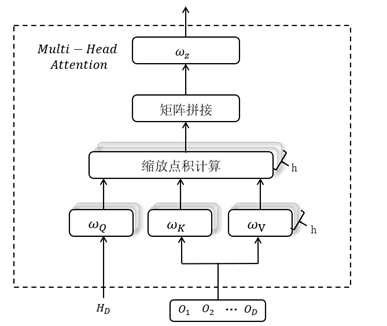点积注意力Pytorch源码：


self.w_q = nn.Linear(ARGS.hidden_dim * (ARGS.bidirect + 1) * ARGS.n_layers, ARGS.dim_k, bias=False)
self.w_k = nn.Linear((ARGS.bidirect + 1) * ARGS.hidden_dim, ARGS.dim_k, bias=False)
self.w_v = nn.ModuleList([
nn.Linear((ARGS.bidirect + 1) * ARGS.hidden_dim, ARGS.dim_v, bias=False)
])

self.w_z2 = nn.Linear(ARGS.num_heads * ARGS.dim_v, ARGS.dim_v, bias=False)

def MultiAttention1(self, lstm_out, h_n):
batch_size, Doc_size, dim = lstm_out.shape
x = []
for i in range(h_n.size(0)):
x.append(h_n[i, :, :])
hidden = torch.cat(x, dim=-1)
key = self.w_k(lstm_out).reshape(batch_size, Doc_size, ARGS.num_heads, dk).transpose(1, 2)
value = [wv(lstm_out).transpose(1, 2) for wv in self.w_v]  # value: n* [batch_size, dim_v, Doc_size]
weights = torch.matmul(key, q_n).transpose(0, 1) / sqrt(dk)  # weights: [n, batch_size, Doc_size, 1]
soft_weights = F.softmax(weights, 2)
out = [torch.matmul(v, w).squeeze() for v,w in zip(value,soft_weights)]
# out[i]:[batch_size, dim_v, Doc_size] × [batch_size, Doc_size, 1] -> [batch_size, dim_v]
# out: [batch_size, dim] * n
out = torch.cat(out, dim=-1)
out = self.w_z2(out)
return out, soft_weights.data  # out : [batch_size, dim_v]



2. 加法注意力：Bahdanau 提出的加法注意力
将最后时间步的隐藏状态 H D H_D 和各时间步输出 O t O_t 拼接作为Query，各时间步输出 O t O_t 线性变换后作为Value，线性变换矩阵 ω k ω_k 作为Key，Query和Value相乘后作为结果矩阵z，时刻t有如下公式：
Q t = ω q ( O t + H D ) Q_t=ω_q(O_t+H_D)
V t = ω v O t V_t=ω_vO_t
z t = t a n h ( Q t ω k ) V t z_t=tanh(Q_tω_k)V_t
结果矩阵为 z = [ z 1 , z 2 , . . . , z t , . . . , z D ] z=[z_1,z_2,...,z_t,...,z_D]
若为多头自注意力，则进行h次操作后获得多个结果矩阵后拼接再做一次线性变换作为输出，如下图：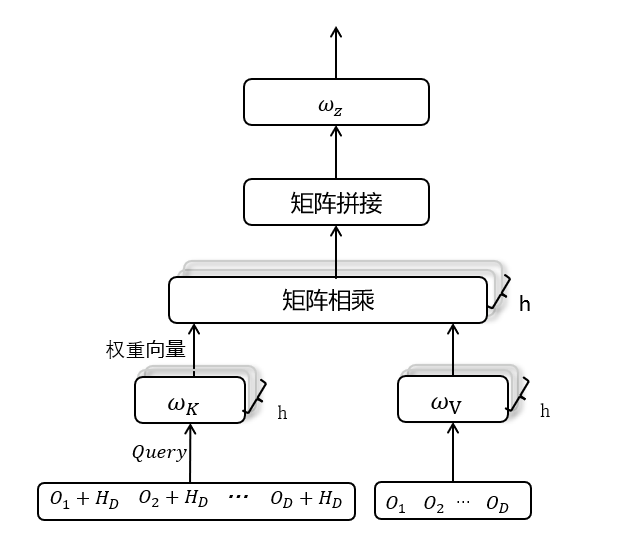加法注意力Pytorch源码：

	    self.w_v = nn.ModuleList([
nn.Linear((ARGS.bidirect + 1) * ARGS.hidden_dim, ARGS.dim_v, bias=False)
])
self.w_z = nn.Linear(ARGS.num_heads * ARGS.dim_v, ARGS.dim_v, bias=False)

self.w_q = nn.Linear((ARGS.bidirect + 1) * ARGS.hidden_dim * (ARGS.n_layers + 1), ARGS.dim_k, bias=True)

self.w_k_Mul = nn.Linear(ARGS.dim_k // ARGS.num_heads, 1, bias=False)

def MultiAttention4(self, lstm_out, h_n):
batch_size, Doc_size, dim = lstm_out.shape
x = []
for i in range(h_n.size(0)):
x.append(h_n[i, :, :])
hidden = torch.cat(x, dim=-1).unsqueeze(dim=-1)
ones = torch.ones(batch_size, 1, Doc_size).to(device)
hidden = torch.bmm(hidden, ones).transpose(1, 2)

# 对lstm_out和hidden进行concat
h_i = torch.cat((lstm_out, hidden), dim=-1)

# 分头，即，将h_i和权值矩阵w_q相乘的结果按列均分为n份，纬度变化如下：
# [batch_size, Doc_size, num_directions*hidden_dim*(1+n_layer)] -> [batch_size, Doc_size, dim_k]
# ->[batch_size, Doc_size, n, dk] -> [batch_size, n, Doc_size, dk]
query = self.w_q(h_i).reshape(batch_size, Doc_size, ARGS.num_heads, dk).transpose(1, 2)
query = torch.tanh(query)  # query: [batch_size, n, Doc_size, dk]

# 各头分别乘以不同的key，纬度变化如下：
# [batch_size, n, Doc_size, dk] * [batch_size, n, dk, 1]
# -> [batch_size, n, Doc_size, 1] -> [batch_size, n, Doc_size]
weights = self.w_k_Mul(query).transpose(0, 1) / sqrt(dk)  # weights: [n, batch_size, Doc_size, 1]
value = [wv(lstm_out).transpose(1, 2) for wv in self.w_v]  # value: n* [batch_size, dim_v, Doc_size]
soft_weights = F.softmax(weights, 2)
# value:[batch_size, dim, Doc_size]
out = [torch.matmul(v, w).squeeze() for v, w in zip(value, soft_weights)]

# out[i]:[batch_size, dim, Doc_size] × [batch_size, Doc_size, 1] -> [batch_size, dim]
# out: [batch_size, dim] * n
out = torch.cat(out, dim=-1)
# out: [batch_size, dim * n]
# print(out.size())
out = self.w_z(out)  # 做一次线性变换，进一步提取特征
return out, soft_weights.data  # out : [batch_size, hidden_dim * num_directions]



Transformer中指出，在高维度的情况下加法注意力的精度优于点积注意力，但是可以通过乘以缩放因子 1 / d k 1/\sqrt{d_k} 抵消这种影响:
以下是论文的3.2.1小节的原文翻译：
最常用的两个注意力函数是加法注意力和点积（多重复制）注意力。点积注意与我们的算法相同，只是比例因子为 1 / d k 1/\sqrt{d_k} 。加法注意力利用一个具有单个隐层的前馈网络来计算相容函数。虽然两者在理论复杂度上相似，但由于可以使用高度优化的矩阵乘法码来实现，因此在实践中，点积注意力速度更快，空间效率更高。
对于较小的 d k d_k ，这两种机制效果相近，对于较大的 d k d_k 值，加法注意力优于点积注意力。我们怀疑，对于较大的 d k d_k 值，点积在数量级上增长很大，从而将softmax函数推到梯度非常小的区域。为了抵消这种影响，我们将点积缩放 1 / d k 1/\sqrt{d_k}

## 5.结语

本文内容主要参考自下述三篇论文以及知乎博文，并对其进行理解和整理。本人也并未完全掌握自注意力机制，想要完全掌握自注意力机制建议阅读这三篇论文的原文。

有错误欢迎指正！

需要源码可私信我哦^ ^

 Mnih V, Heess N, Graves A, et al. Recurrent Models of Visual Attention. arXiv preprint, arXiv: 1406.6247 [ cs. CL] 2014.
 Vaswani A, Attention Is All You Need, arXiv preprint, arXiv: 1706.03762 [cs.CL] 2017.
 Bahdanau D, Cho K, Bengio Y. Neural Machine Translation by Jointly Learning to Align and Translate[J]. Computer Science, 2014.

展开全文神经网络
• 为提高行人交互中轨迹预测速度、精度与模型可解释性，提出了一种基于社会注意力机制的GAN模型。首先，定义了一种新型社会关系，对行人间的影响进行社会关系建模，设计了基于注意力机制的网络模型，提高了网络预测...生成对抗网络
• Attention.zip文件中总结了几种关于注意力机制的代码，有keras和tensorflow，还有PyTorch框架的
• 自注意力机制(Self-attention) 背景 最近，学了好多东西，今天看了一下李飞飞老师讲解的自注意力机制，因此在这记录一下，以供日后复习，同时自己学习消化知识也好。 综述 一般来说，模型的输入输出有三种： ...

# 自注意力机制(Self-attention)

## 背景

最近，学了好多东西，今天看了一下李宏毅老师讲解的自注意力机制，因此在这记录一下，以供日后复习，同时自己学习消化知识也好。

### 综述

一般来说，模型的输入输出有三种：

• N个输入，经过模型计算后，输出N个结果，也就是对输入向量进行计算，从而得到每个向量对应的输出值。

• N个输入，送入模型进行计算，最终得到一个结果。这就是平时常见的比如，文本分类、情感分析等。

• 任意个输入，输出任意个输出。这种在生活中也较为常见，比如机器翻译，对于一句话，不同的语言会包含不同个词。

此文先讲讲第一种，也就是说N个输入，得出N个结果，这种模型一般形式如下图所示。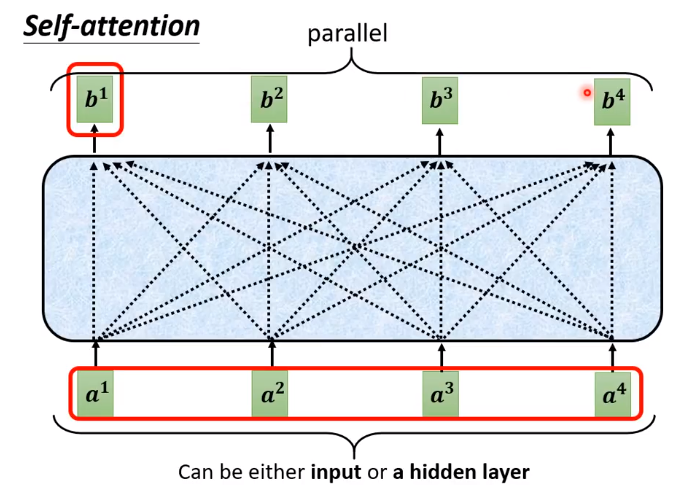在图中可以看到，a表示对输入数据进行处理之后，作为self-attention的输入，从而得到b。其中，在得到每个b的过程中，都会考虑到输入的每个元素，包括a1, a2, a3, a4。这样对于输出的结果更合理。

对于中间那一块深色的模块，就是自注意力机制的核心。在注意力机制中，首先需要计算注意力得分，比如：在进行计算第一元素时，需要考虑其他三个元素的情况，在计算第一个元素时，分别考虑其他每个元素的权重，给每个元素打分，重要性越大则分数越高。

其计算得分的流程如图下所示。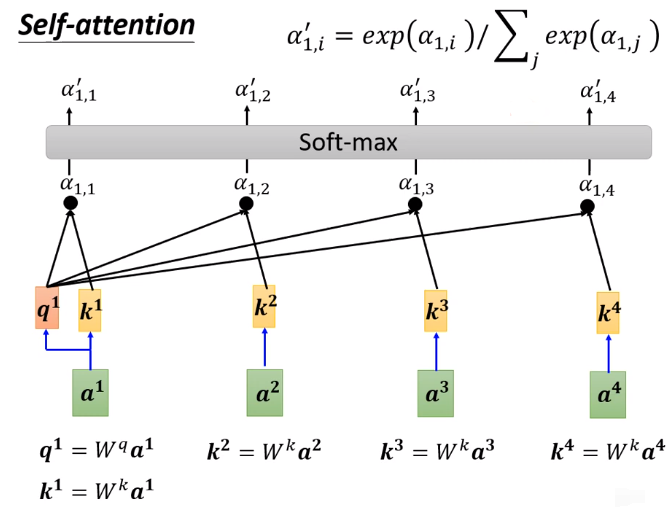在上图中可以得到，自注意力机制在计算第一个元素时流程为：

1. 首先，给输入向量每个元素，分别乘以两个举证Wq和Wk，从而计算出q1和ki。

2. 然后，将q1和ki分别相乘，得到每个元素的分数。

3. 为了得到更好的结果，对每个分数进行一次soft-max。

最后，将每个分数按图上公式进行计算，得到输出在计算每个元素时，对应考虑其他元素的注意力分数。

在得到分数之后，可以通过以下图得到b，具体如下图所示。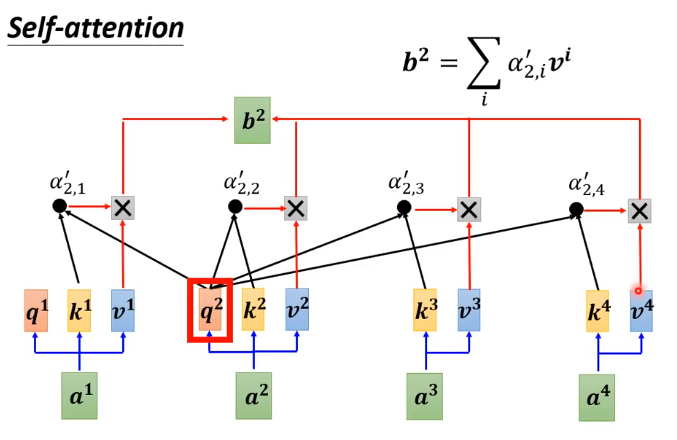在上图中，结合计算注意力得分的图，可以看出，在得出注意力分数之后，在将分数和vi进行相乘之后，再进行加权求和，即可得到b1。用同样的分数可以的b2,b3,b4。

### 矩阵形式

为了方便计算，一般会通过矩阵形式来计算，如图所示。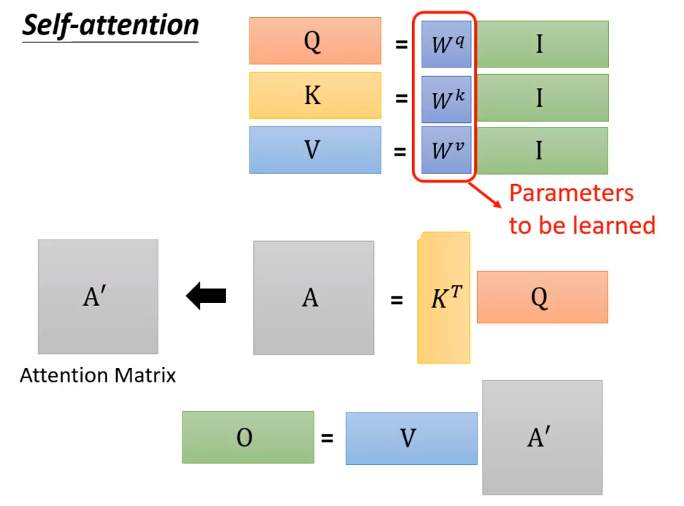在上图中可以清楚的看到，在进行自注意力中用矩阵形式进行计算过程。

其中I为输入向量进行拼接后的举证，Q、K、V矩阵为每个输入向量对应的q、k、v进行拼接后的矩阵。

展开全文• 回归预测 | MATLAB实现TPA-LSTM(时间注意力注意力机制长短期记忆神经网络)多输入单输出（完整源码和数据） 多输入单输出,运行环境MATLAB2020b及以上，运行主文件TPAMain.m即可。
• 为了同时捕获到空间和时间上的依赖, 提出了一个新的神经网络: 基于注意力机制的时空图卷积网络 (A-TGCN). TGCN网络模型用于捕获交通数据中的动态时空特性与相关性, 采用注意力机制来增强每个A-TGCN层中关键节点的...
• 针对长 短期记忆网络（LSTM） 在行人轨迹预测问题中孤立考虑单个行人，且无法进行多种可能性预测的问题，提出基于注意力机制的行人轨迹预测生成模型（AttenGAN），来对行人交互模式进行建模和概率性地对多种合理可能...
• 融合图卷积神经网络和注意力机制的PM2.5小时浓度多步预测.pdf
• matlab出图，运行保障，代码有详细说明，适用初学者。
• 人工智能-基于注意力机制的神经网络预测模型.pdf
• Keras注意机制 在Keras中为以下层实现了简单的关注机制： 密集（注意2D块） LSTM，GRU（注意3D块） 示例：注意块 致密层 inputs = Input(shape=(input_dims,)) attention_probs = Dense(input_dims, activation=...Python
• 摘 要 一 一 经 有助 决策者判断当前的 济 势 另 方 对 票价格的准确预测 方面 于 形 股 于 但是 进 确 非常 难的 因为 获 收益 对 票价格 行准 预测是 困 面有助 投资者 取 股 游 模 股 是 线性且 机 走的时间序列 ...
• 本文提出一种基于自注意力机制的局部与全局特征融合的评分预测算法（Rating Prediction Algorithm Based on Self-Attention Mechanism and Fusion of Local & Global Features，AMFL&GRec） 框架主要分为四...算法 人工智能 数据挖掘
• 基于注意力机制的GRU神经网络安全态势预测方法.pdf
• 时隔半年多，毕设男孩终于重操旧业，回到了 LSTM进行时间序列预测和异常检测的路上。...本次我们要进行的是 使用 注意力机制 + LSTM 进行时间序列预测，项目地址为Keras Attention Mechanism 首先我们把它git...lstm attention
• 基于自注意力机制的双向门控循环单元和卷积神经网络的芒果产量预测.pdf
• 方程 1: 两个随机变量X和Y的协方差 CNN中的自注意力机制 图3: CNN中的自注意力机制 为了实现对每个像素级预测的全局参考，Wang等人在CNN中提出了自我注意机制(图3)。他们的方法是基于预测像素与其他像素之间的...卷积 算法 python 计算机视觉 机器学习
• 回归预测 | MATLAB实现Attention-LSTM(注意力机制长短期记忆神经网络)多输入单输出（完整源码和数据） 多输入单输出,运行环境MATLAB2020b及以上。
• 机器翻译 机器翻译（MT）：将一段文本从一种语言自动翻译为另一种语言，用神经网络解决这个问题通常称为神经机器翻译（NMT）。 主要特征：输出是单词序列而不是单个...注意力机制 在“编码器—解码器（seq2seq）”⼀节
• 基于注意力机制的电信用户离网预测算法，杨晨，朱新宁，随着电信市场用户增速的逐渐放缓，电信市场竞争趋于白热化，电信用户更换运营商的情况日益普遍。获取新用户的费用远远超过挽回潜人工智能
• 本文主要参考开源资料对Attention注意力机制进行总结，注意力机制本质上与人类对外界事物的观察机制相似。以下是相关学习资料推荐： 深度学习中的注意力机制_CSDN大数据-CSDN博客 目前主流的attention方法都有哪些？...深度学习 神经网络 attention
• 针对时序数据特征，提出一种基于注意力机制的卷积神经网络（CNN）联合长短期记忆网络（LSTM）的神经网络预测模型，融合粗细粒度特征实现准确的时间序列预测。该模型由两部分构成：基于注意力机制的CNN，在标准CNN...
• 注意力机制放到这里，以后会用到。 练习题放在最前面： 关于Transformer描述正确的是： 在训练和预测过程中，解码器部分均只需进行一次前向传播。 Transformer 内部的注意力模块均为注意力模块。 解码器部分在......